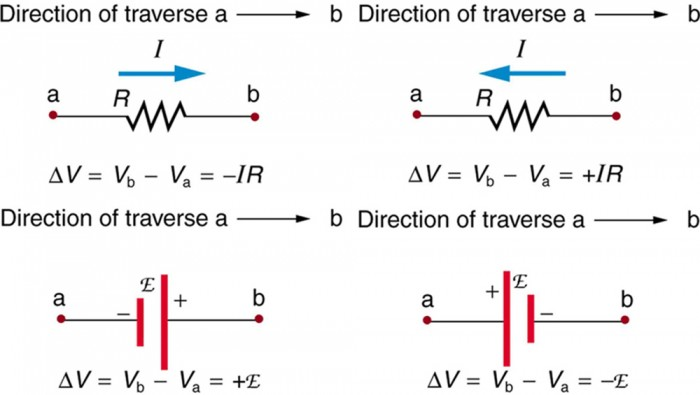# Kirchoff's law and its application.Kirchhoff’s Laws

• What are Kirchhoff’s Laws?

Kirchhoff’s laws govern the conservation of charge and energy in electrical circuits.

• Kirchhoff’s Laws:

1. The junction rule

2. The closed-loop rule

Junction Rule

• “At any node (junction) in an electrical circuit, the sum of currents flowing into that node is equal to the sum of currents flowing out of that node, or: The algebraic sum of currents in a network of conductors meeting at a point is zero”.

• The sum of currents entering the junction are thus equal to the sum of currents leaving. This implies that the current is conserved (no loss of current).

Σ𝐼𝑖𝑛= Σ𝐼𝑜𝑢𝑡

Close Loop Rule

• The principles of conservation of energy imply that the directed sum of the electrical potential differences (voltage) around any closed circuit is zero.

Σ∆𝑉𝑐𝑙𝑜𝑠𝑒 𝑙𝑜𝑜𝑝= 0

Conventions for Loop Rule

• The following conventions apply for determining the sign of delta V across circuit elements. The travel direction is the direction that we choose to proceed around the loop.Procedure for Applying Rules

1. Assume all voltage sources and resistances are given. (If not label them V1, V2 ..., R1, R2 etc)

2. Label each branch with a branch current. (I1, I2, I3 etc)

3. Apply junction rule at each node.

4. Applying the loop rule for each of the independent loops of the circuit.

5. Solve the equations by substitutions.